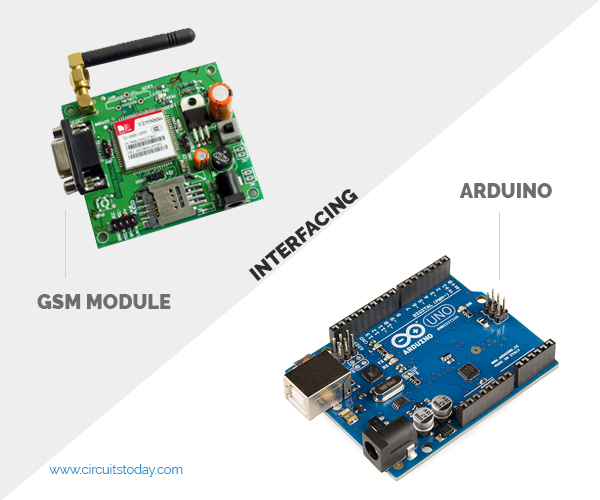# Scrolling Text Display on 8×8 LED Matrix using Arduino

In this tutorial we are going to design an 8×8 LED Matrix Scrolling Display using Arduino Uno, which will show scrolling alphabets.

8×8 LED Matrix contains 64 LEDs (Light Emitting Diodes) which are arranged in the form of a matrix, hence the name is LED matrix. We are going to make this Matrix by soldering these 64 LEDs on to the perfboard or DOT PCB. The LEDs can be of any color, choose the ones which are available with you. Then we will write a program for Arduino to control these 64 LEDs matrix. The UNO, according to program, powers appropriate LEDs to show characters in scrolling fashion.

### Components Required:

• Arduino Uno
• 64 LEDs
• Perfboard with other soldering tools
• 1KΩ resistor (8 pieces)
• Power Supply (5v)### Circuit and Working Explanation:

There are 64 LEDs arranged in a matrix form. So we have 8 columns and 8 rows. Over those rows and columns, all the positive terminals in a row are brought together. For each row, there is one Common Positive Terminal for all 8 LED in that row. It is shown in below figure,So for 8 rows we have 8 common positive terminals. Consider the first row, as seen in the figure, 8 LEDs from D57 to D64 have a common positive terminal and are denoted by ‘POSITIVE0’. Now if we want glow one or all LEDs in the first ROW of matrix, then we should power the PIN0 of LED Matrix. Likewise if we want to glow any LED (or all) in any ROW then we need to power the corresponding Common Positive Terminal Pin of that respective Row.